## Example Questions

### Example Question #1 : How To Add Fractions

Jesse has a large movie collection containing X movies. 1/3 of his movies are action movies, 3/5 of the remainder are comedies, and the rest are historical movies. How many historical movies does Jesse own?

(3/9)*X

(2/5)*X

(4/15)*X

(7/12)*X

(11/15)*X

(4/15)*X

Explanation:

1/3 of the movies are action movies. 3/5 of 2/3 of the movies are comedies, or (3/5)*(2/3), or 6/15. Combining the comedies and the action movies (1/3 or 5/15), we get 11/15 of the movies being either action or comedy. Thus, 4/15 of the movies remain and all of them have to be historical.

### Example Question #2 : How To Add Fractions

If x = 1/3 and y = 1/2, find the value of 2x + 3y.

13/6

2

5/6

6/5

1

13/6

Explanation:

Substitute the values of x and y into the given expression:

2(1/3) + 3(1/2)

= 2/3 + 3/2

= 4/6 + 9/6

= 13/6

### Example Question #3 : How To Add Fractions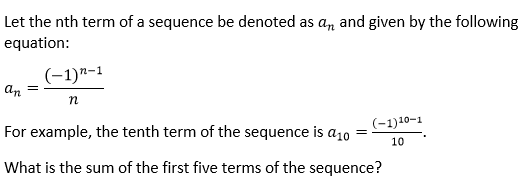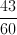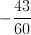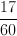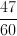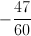Explanation: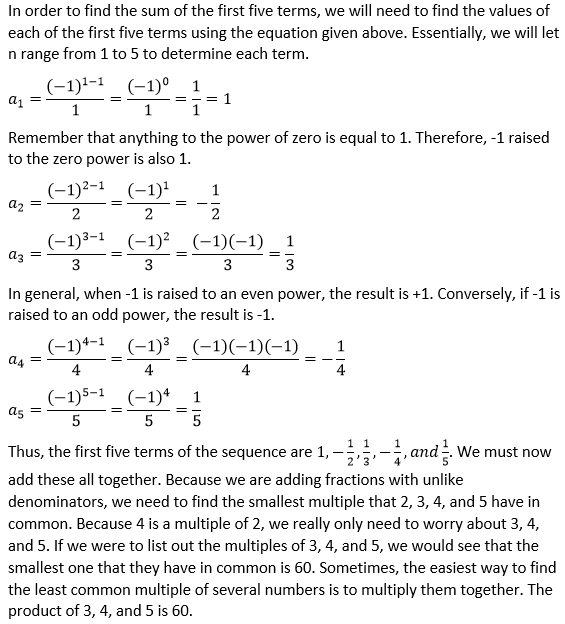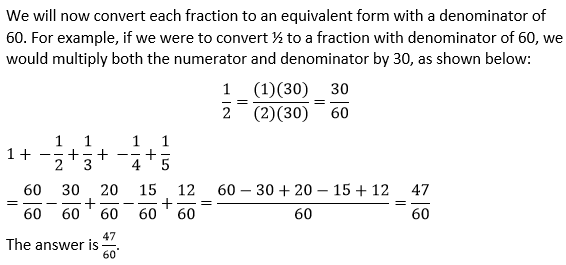### Example Question #4 : How To Add Fractions

What is the solution, reduced to its simplest form, for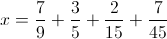?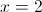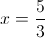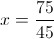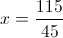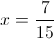Explanation: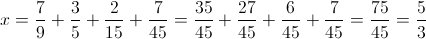### Example Question #1 : Operations

What is the result of addingofto?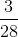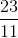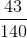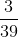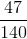Explanation:

Let us first get our value for the percentage of the first fraction. 20% of 2/7 is found by multiplying 2/7 by 2/10 (or, simplified, 1/5): (2/7) * (1/5) = (2/35)

Our addition is therefore (2/35) + (1/4). There are no common factors, so the least common denominator will be 35 * 4 or 140. Multiply the numerator and denominator of 2/35 by 4/4 and the numerator of 1/4 by 35/35.

This yields:

(8/140) + (35/140)  = 43/140, which cannot be reduced.

### Example Question #6 : How To Add Fractions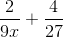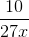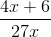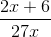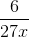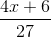Explanation:

Find the least common denominator to solve this problem

Multiply 27 with, and multiply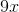with 3 to obtain common denominators.

Convert the fractions.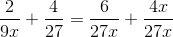Combine the terms as one fraction.

The answer is:### Example Question #7 : How To Add Fractions

Solve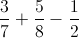.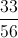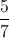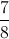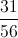Finding the common denominator yields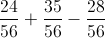. We can then evaluate leaving.